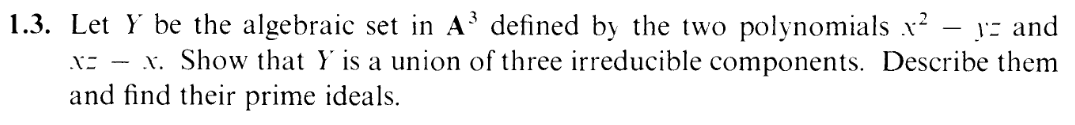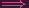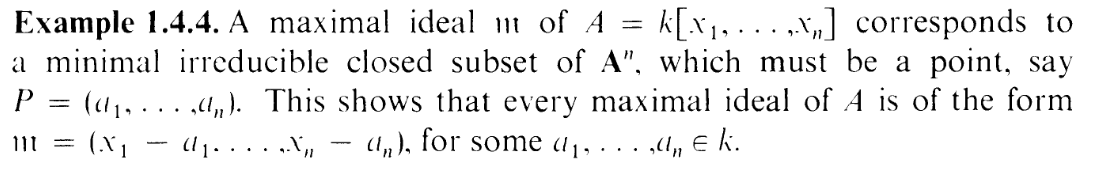I.1.3

11/29/2020I gave this sucker a shot on the eve of Thanksgiving, struggled, got frustrated, then gave up for the holiday weekend. So much for the 1 exercise every 2 days plan I had worked out in 1.1b, eh?

I did not spend the weekend well, I'll tell you that. My motivation to do stuff got sapped by sappy realizations about the course of all my efforts. To what end, my friends? Blah blah blah. Then, sitting here at the foot of the new week was an obligation to do exercise 1.3. Why did I start this blog? So that I wouldn't embarass myself by posting 3 exercises and then pathetically dropping off. Well, here I am. The foot is here and it's time to start licking.

So here's the deal: my Thanksgiving eve approach was overcomplicated. Basically I was trying to do it via some overcomplicated abstract algebra logic. But this exercise is actually high school level stuff. Really. You just have to rephrase the zero set.

They gave us
Y = Z(x2 - yz,xz - x).

Basically, we need
x2 - yz = 0  and xz - x = 0 .
The latter equation is actually just

x(z - 1) = 0

So we have two cases.

CASE 1: x = 0
What happens to the other equation here?
x2 - yz = 0- yz = 0
Which itelf splits into 2 cases
CASE 1A: y = 0
CASE 1B: z = 0

I.e. case 1 as a whole is x = 0,y = 0 (the z axis) or x = 0,z = 0 (the y axis).

CASE 2: z - 1 = 0
I.e. z = 1.
What happens to equation  in this case? It becomes the parabola:
y = x2

So case 2 as a whole is a parabola on the z = 1 plane.

In total, we have
x = 0,y = 0 OR x = 0,z = 0 OR z = 1,y = x2

I.e. we can rewrite Y as
Y = Z(x,y) Z(x,z) Z(y - x2,z - 1)

(x,y),(x,z) are maximal ideals according to the book (Example 1.4.4):and therefore they're prime.

And (y - x2,z - 1) is prime thanks to the same sort of Euclidean division argument from the last exercise.

So... yeah... that's it. 3 days of stalling for that.

Like last time's exercise, I finished the solution without cussing. But this time, to commemorate my fetish being well out of the bag, I'll conclude with FEET FEET FEET FEET FEET FEET FET FEET FEET FEET FEET FEET FEET FEET FEET FEET FEET FEET FEET EET FEET FEET FEET FEET FEET FEET FEET FET FEET FEETFEFET FEET FEET FEET FEET FEET FEETF FEET FEET FEET FEET FET FEET FEET FEET FEET FEET FEET FEET FEET FEET FEET FEET FEET FEETE FEET FEET FEET FEET FEET FEET FEET FEET FEET FEET FEEET FEET FEET FEET FEET FEET FEET FEETF EET FETT FEET FET FEET FEET FEET FEET FET FEET FEET FEET FEET FEET FEET FEET FEET FEET FEET FEET FEET FEET FEET FEET FEET FEET FEET FEET FEET FEET FEET FEETT FET EET FEET FEET FEET FEET FEET FEET FEET EET FEET AHH FEET